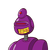# The radius of Circle A is 7 ft. The radius of Circle B is 3 ft greater than the radius ofCircle A. The radius of Circle C is

The radius of Circle A is 7 ft. The radius of Circle B is 3 ft greater than the radius of
Circle A. The radius of Circle C is 3 ft greater than the radius of Circle B. The radius of Circle D is 2 ft
less than the radius of Circle C. What is the area of each circle? How many times greater than the
area of Circle A is the area of Circle D?​

### 1 thought on “The radius of Circle A is 7 ft. The radius of Circle B is 3 ft greater than the radius of<br />Circle A. The radius of Circle C is”

1.Step-by-step explanation:

Ar of circle

A= 49π

B=100π

C=169π

D=121π

Ar of circle A is less than Ar of circle D